Want to share your content on R-bloggers? click here if you have a blog, or here if you don't.

In the previous post (here) discussing forecasts of actuarial quantities, I did not mention much how to forecast the temporal component in the Lee-Carter model. Actually, many things can be done. Consider here some exponential smoothing techniques (see here for more details). For instance, with a simple model, with an additive error, no trend and no seasonal component,or equivalently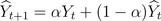Then, and if we want a confidence interval

```> (ETS.ANN=ets(Y,model="ANN"))
ETS(A,N,N)

Call:
ets(y = Y, model = "ANN")

Smoothing parameters:
alpha = 0.7039

Initial states:
l = 75.0358

sigma:  11.4136

AIC     AICc      BIC
941.0089 941.1339 946.1991
> plot(forecast(ETS.ANN,h=100))```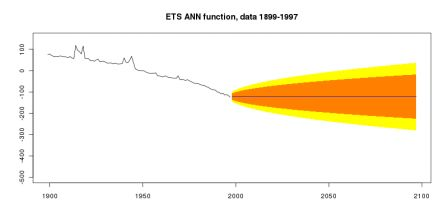Here, a natural idea will probably be to take into account a linear trend on the series, i.e.where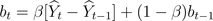then the forecast we make isi.e.

```ETS.AAN=ets(Y,model="AAN")
plot(forecast(ETS.AAN,h=100))```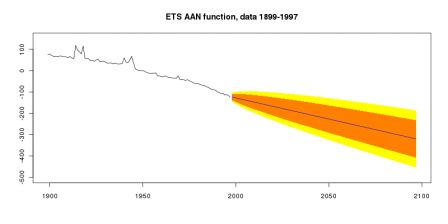It is also possible to ask for an “optimal” exponential smoothing model (without any specification)

```> ETS
ETS(A,A,N)

Call:
ets(y = Y)

Smoothing parameters:
alpha = 0.6107
beta  = 1e-04

Initial states:
l = 74.5622
b = -1.9812

sigma:  11.0094

AIC     AICc      BIC
937.8695 938.2950 948.2500
> plot(forecast(ETS,h=100))```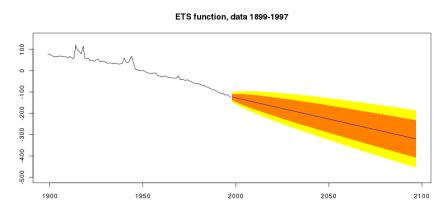Another idea can be to consider some autoregressive integrated moving average models (ARIMA), here with a linear trendFor instance, if we want only the integrated component, i.e.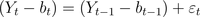then the code is

```> ARIMA010T=Arima(Y,order=c(0,1,0),include.drift=TRUE)
Series: Y
ARIMA(0,1,0) with drift

Call: Arima(x = Y, order = c(0, 1, 0), include.drift = TRUE)

Coefficients:
drift
-2.0337
s.e.   1.1904

sigma^2 estimated as 138.9:  log likelihood = -380.8
AIC = 765.6   AICc = 765.73   BIC = 770.77
> plot(forecast(ARIMA010T,h=100))```But note that any kind of ARIMA model can be considered, e.g. integrated with an autoregressive component (here of order 1)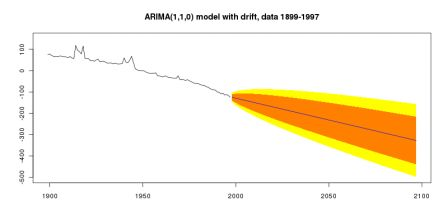or with also a moving average component (again of order 1)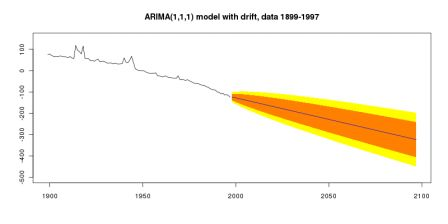Actually, note that, once again, we can ask for an automatic selection of the model,

```> (autoARIMA=auto.arima(Y,allowdrift=TRUE))
Series: Y
ARIMA(0,1,1) with drift

Call: auto.arima(x = Y, allowdrift = TRUE)

Coefficients:
ma1    drift
-0.3868  -2.0139
s.e.   0.0970   0.6894

sigma^2 estimated as 122.3:  log likelihood = -374.65
AIC = 755.29   AICc = 755.55   BIC = 763.05
> plot(forecast(autoARIMA,h=100))```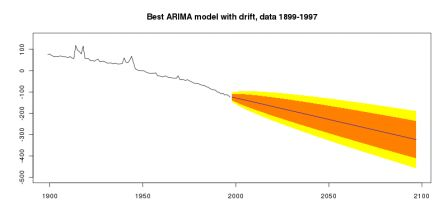Finally, it is possible to use also also some specific functions of the package, for instance to consider a random walk with a drift,

```RWF=rwf(Y,h=100,drift=TRUE)
plot(RWF)```or Holt model (with a trend)

```HOLT=holt(Y,h=100,damped=TRUE)
plot(HOLT)```And if all that is not enough, it is also possible to go further by changing the size of the series we use to fit the model. A question that naturally arises is the treatment of wars in our model: shouldn’t we assume that the forecast should be based only on the last 50 years (and exclude wars from our analysis) ? In that case, for instance, the exponential smoothing technique gives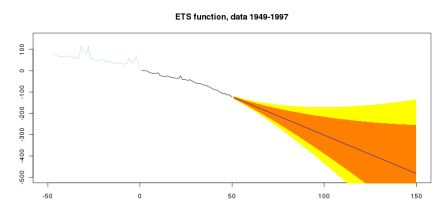while the ARIMA procedure returns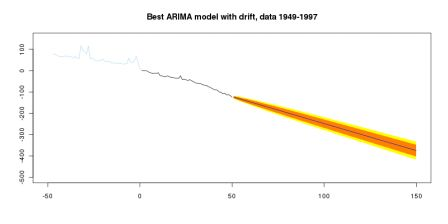And finally, with the Holt technique, we haveSo, it looks like we have a lot of techniques that can be used to provide a forecast for the temporal component in the Lee-Carter model,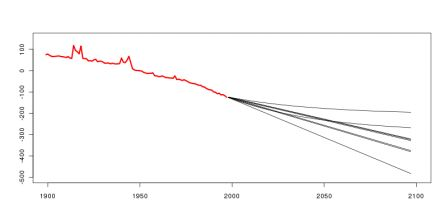All those estimators can be used to estimate annuities of insurance contracts (as here),

```BASEB=BASEB[,1:99]
BASEC=BASEC[,1:99]
AGE=AGE[1:99]
library(gnm)
D=as.vector(BASEB)
E=as.vector(BASEC)
A=rep(AGE,each=length(ANNEE))
Y=rep(ANNEE,length(AGE))
base=data.frame(D,E,A,Y,a=as.factor(A),
y=as.factor(Y))
LC2=gnm(D~a+Mult(a,y),offset=log(E),
family=poisson,data=base)

A=LC2\$coefficients+LC2\$coefficients[2:99]
B=LC2\$coefficients[100:198]
K0=LC2\$coefficients[199:297]
Y=as.numeric(K01)
K1=c(K0,forecast(ets(Y),h=120)\$mean)
K2=c(K0,forecast(auto.arima(Y,allowdrift=TRUE),h=120)\$mean)
K3=c(K0,rwf(Y,h=120,drift=TRUE)\$mean)
K4=c(K0,holt(Y,h=120,drift=TRUE)\$mean)
K5=c(K0,forecast(ets(Y[50:99]),h=120)\$mean)
K6=c(K0,forecast(auto.arima(Y[50:99],allowdrift=TRUE),h=120)\$mean)
K7=c(K0,rwf(Y[50:99],h=120,drift=TRUE)\$mean)
K8=c(K0,holt(Y[50:99],h=120,drift=TRUE)\$mean)

MU=matrix(NA,length(A),length(K1))
MU1=MU2=MU3=MU4=MU5=MU6=MU7=MU8=MU

for(i in 1:length(A)){
for(j in 1:length(K1)){
MU1[i,j]=exp(A[i]+B[i]*K1[j])
MU2[i,j]=exp(A[i]+B[i]*K5[j])
MU3[i,j]=exp(A[i]+B[i]*K3[j])
MU4[i,j]=exp(A[i]+B[i]*K4[j])
MU5[i,j]=exp(A[i]+B[i]*K5[j])
MU6[i,j]=exp(A[i]+B[i]*K6[j])
MU7[i,j]=exp(A[i]+B[i]*K7[j])
MU8[i,j]=exp(A[i]+B[i]*K8[j])
}}

t=2000
x=40
s=seq(0,98-x-1)

Pxt1=cumprod(exp(-diag(MU1[x+1+s,t+s-1898])))
Pxt2=cumprod(exp(-diag(MU2[x+1+s,t+s-1898])))
Pxt3=cumprod(exp(-diag(MU3[x+1+s,t+s-1898])))
Pxt4=cumprod(exp(-diag(MU4[x+1+s,t+s-1898])))
Pxt5=cumprod(exp(-diag(MU5[x+1+s,t+s-1898])))
Pxt6=cumprod(exp(-diag(MU6[x+1+s,t+s-1898])))
Pxt7=cumprod(exp(-diag(MU7[x+1+s,t+s-1898])))
Pxt8=cumprod(exp(-diag(MU8[x+1+s,t+s-1898])))

r=.035
m=70
h=seq(0,21)
V1=1/(1+r)^(m-x+h)*Pxt1[m-x+h]
V2=1/(1+r)^(m-x+h)*Pxt2[m-x+h]
V3=1/(1+r)^(m-x+h)*Pxt3[m-x+h]
V4=1/(1+r)^(m-x+h)*Pxt4[m-x+h]
V5=1/(1+r)^(m-x+h)*Pxt5[m-x+h]
V6=1/(1+r)^(m-x+h)*Pxt6[m-x+h]
V7=1/(1+r)^(m-x+h)*Pxt7[m-x+h]
V8=1/(1+r)^(m-x+h)*Pxt8[m-x+h]```

Hence, here the difference is significant,

```> M=cbind(V1,V2,V3,V4,V5,V6,V7,V8)
> apply(M,2,sum)
V1       V2       V3       V4       V5       V6       V7       V8
4.389372 4.632793 4.406465 4.389372 4.632793 4.468934 4.482064 4.632793```

or graphically,